# Non-homogeneous Recurrence Relations

#### (A new question of the week)

A recent question asked us to find errors in solving recurrence relations by the method of undetermined coefficients. We’ll see several things that can go wrong, and correct some misunderstandings.

## 1: First order recurrence

Here is the initial question, submitted by Aaron in late February:

Which step I am doing wrong?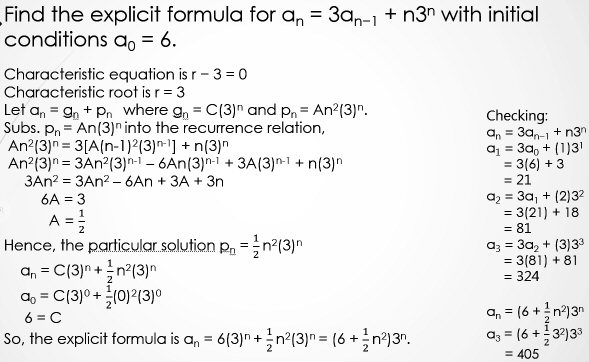We have mentioned recurrence relations and recursive sequences in Introducing the Fibonacci Sequence and Fibonacci Word Problems I: Basic; in particular, we saw there how to start with the recursive definition of that sequence, $$F_0 = 1\\ F_1 = 1\\ F_n = F_{n-1} + F_{n-2}\text{ for }n>1$$ (also called a recurrence relation), where each term is found using previous terms, and solve it to find an explicit formula, $$F_n = \frac{\phi^n-(1-\phi)^n}{\sqrt{5}}$$

This is a second-order relation, in which one term is related to the two preceding terms. A well-known first-order relation is the factorial, which can be defined by $$F_0 = 1\\ F_n = nF_{n-1}\text{ for }n>0$$ Note that in this case, not only the preceding term, but also the index n itself, is part of the equation. This will be true of those we examine here.

A linear recurrence relation (with constant coefficients) is one where the equation has the form $$u_n=a_1u_{n-1}+a_2u_{n-2}+\dots+a_{k-1}u_{n-(k-1)}+a_ku_{n-k}+b$$

If $$b=0$$, the recurrence is called homogeneous, and there is a simple way to solve it. We write the equation as $$u_n-a_1u_{n-1}-a_2u_{n-2}-\dots-a_{k-1}u_{n-(k-1)}-a_ku_{n-k}=0$$ and form the characteristic equation $$x^k-a_1x^{k-1}-a_2x^{k-2}-\dots-a_{k-1}x-a_k=0$$ The roots of this equation are used to form the solutions.

What we are working with in these problems are non-homogeneous linear recurrences with constant coefficients, where “b” is not only non-zero, but (typically) a function of n; these are solved by first replacing this non-homogeneous term with zero and solving to obtain the general solution $$g_n$$ of the homogeneous equation, and also finding a particular solution $$p_n$$ of the entire recurrence. The solution will be a sum of these, whose coefficients are determined by the initial conditions.

We see Aaron choosing the form $$g_n=C(3)^n$$ and $$p_n=An^2(3)^n$$, where A and C are numerical coefficients to be determined.

I am not fluent in this subject, but chose to answer:

Hi, Aaron.

I first see what could be a simple algebraic error: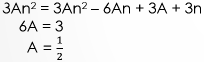In the second line, you have lost the +3A term from the first line. If you solve correctly for A, you would get

6An = 3A + 3n

2An = A + n

A = n/(2A – 1)

Since this is not independent of n, your particular solution doesn’t work.

Properly, you want the two sides to be equivalent functions of n, so you should simplify

6An = 3A + 3n

and then match like terms,

terms in n: 6A = 3

constant terms: 0 = 3A

which is an inconsistent system of equations.

The equations imply that $$A=\frac{1}{2}$$ and $$A=0$$, which can’t both be true! This is a common sign that we started wrong; we’ll be seeing it again!

So the major error appears to be in your choice of the particular solution

pn = An2 3n.

Can you show us what you were taught about selecting a particular solution?

You can get a solution if you instead take

pn = (An2 + Bn)3n.

Try that, unless you were taught something different.

I was looking at online notes trying to find something that covered this particular sort of problem to see what is taught, because if I ever studied this, it was a long time ago! It was hard to find anything that dealt with this level of problem, so I couldn’t be sure how Aaron was being taught. He later sent the material he is learning from, which contains this table: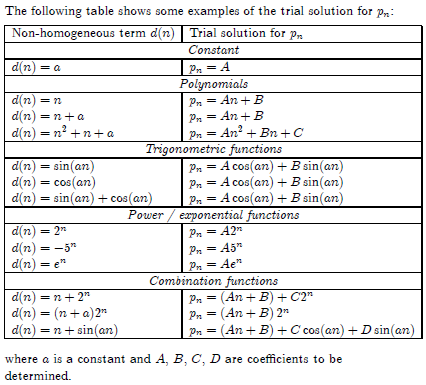Our homogeneous part, $$n3^n$$, fits the next-to-last line, $$a_n=(n+a)2^n$$, with $$a=0$$. Typically, we make a particular solution by combining the form of the non-homogeneous term itself with other terms that might arise from applying the recursion to it, here $$p_n=(An+B)2^n$$. For polynomials, for instance, it is found that a general polynomial of the same degree is needed, not just the single power.

But the problem at hand is a special case, where the base of the exponential in the non-homogeneous term is a root of the characteristic equation, so that $$3^n$$ is already part of the homogeneous solution. They give a simpler example suggesting to multiply by n in order to avoid duplication, and have enough flexibility to solve for the coefficients. No example in my sources or his was quite like this problem.

What Aaron had done was just to use $$An^23^n$$. But perhaps we need either to use a complete polynomial $$(An^2+Bn+C)3^n$$, or just multiply $$(An+B)3^n$$ by n. I’d tried the latter, and it worked.

### Corrected solution

Aaron responded, having tried my suggestion and found that it failed his check:

Dear Dr. Peterson,

Thank you very much for your quick response.

I still cannot find the correct answer. If my answer is correct, I must be able to check it correctly. Please help me again to find out which step is incorrect. Thank you very much for your help.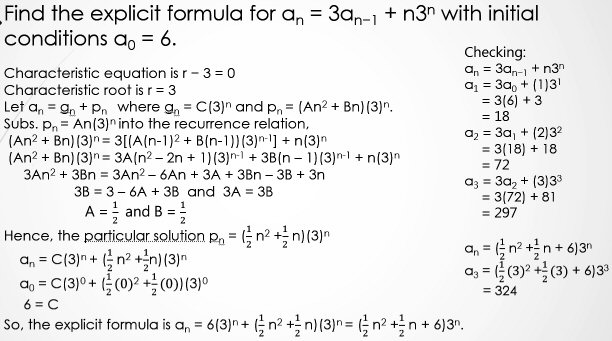Let’s look more closely at his work this time, because it turns out to be correct.

He first used the characteristic root 3 to determine the general solution $$g_n=C3^n$$, where C can be any constant; this will satisfy the homogeneous equation $$a_n=3a_{n-1}$$. Then, as I’d suggested, he chose the particular solution $$p_n=(An^2+Bn)3^n$$, where A and B are constants to be determined from the recurrence. If the base of the exponential term hadn’t been one already found in the general solution, we would have used $$p_n=(An+B)3^n$$, but we have multiplied by n to avoid duplication.

Putting this trial solution into the recurrence relation and dividing everything by $$3^{n-1}$$, he obtained $$3An^2+3Bn=3An^2-6An+3A+3Bn-3B+3n$$ which can be arranged, combining like terms, into $$3An^2+3Bn=3An^2+(-6A+3B+3)n+(3A-3B)$$ Setting coefficients of like terms equal, he gets $$3A=3A\\ 3B=-6A+3B+3\\ 0=3A-3B$$ which he solves to find that $$A=\frac{1}{2}, B=\frac{1}{2}$$. (Observe that he got the same A before, but the presence of B prevents inconsistency.)

Combining the general and particular solutions, he now has $$a_n=C3^n+\left(\frac{1}{2}n^2+\frac{1}{2}n\right)3^n$$ Setting $$a_0=6$$, the equation reduces to $$C=6$$, yielding the solution $$a_n=\left(\frac{1}{2}n^2+\frac{1}{2}n+6\right)3^n$$

I replied:

When you redid the recurrence this time as part of your check, you did it incorrectly, so you are comparing against the wrong numbers. What you had before, and what you wrote this time, are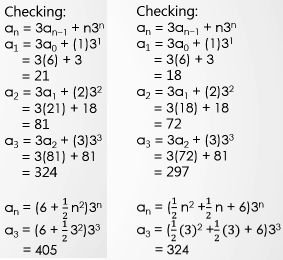This time, you forgot to add 3(6) + 3 to get 21. Your new formula value of 324 was correct all along: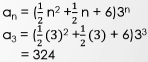It is not uncommon for students to have a correct answer but get the check wrong (or, similarly, to think their answer is wrong because it doesn’t match their book’s answer, which turns out to be wrong).

When I solved the problem earlier, I made a spreadsheet with the actual sequence in one column and the result of my formula in another: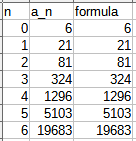So, good work! And don’t forget in the future to check your checks, and doubt your doubts!

A spreadsheet is a good way to check work on recursive sequences. Here, the formula in the second column obtains each term from those before it (cell B4 has “=3*B3+A4*3^A4” yielding 21), while the third column applies the formula we derived (cell C4 has “=(1/2*A4^2+1/2*A4+6)*3^A4”).

## 2: Second order recurrence

Aaron replied with thanks and a new question:

Thank you very much for your quick response and mindless help.

Find the explicit formula for an + 6an−1+ 9an−2 = (-3)n for n ≥ 2 where a0 = 2 and a1 = 3.

What is the particular solution?

I try (An2 + Bn + C)(-3)n or An2(-3)n does not work.

(I assume “mindless” is a typo for some complimentary word, but I can’t think what it is, so I’ve left it!)

Here each term depends on two previous terms. The technique for solving is generally the same, but the characteristic equation is now quadratic, which can result in two roots (in which case we have to add powers of both roots to form a general solution), or in one repeated root, which will be true here. In the latter case, in order to have two terms (from which to solve for two parameters), we multiply a power of the root by n, as we’ll see here.

He quickly wrote again, showing his work:

This seems to work for An2(-3)n, but something for my checking.

Sorry to bother you again.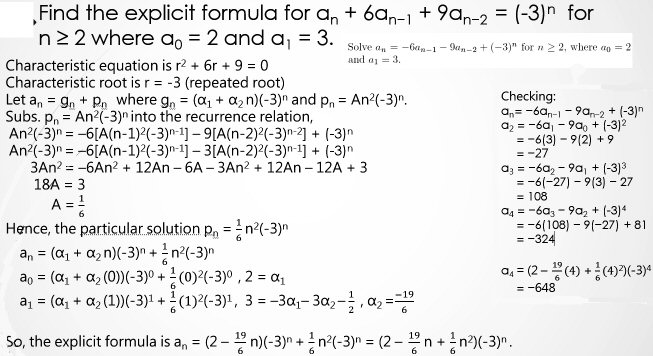(For some reason Aaron has switched from using letters like A and B as constants in the general solution to using Greek alphas; I find the former easier to keep track of, and less likely to be confused with the sequence itself.)

Since we have a repeated root, $$r=-3$$, the general solution is $$g_n=(\alpha_1+\alpha_2n)(-3)^n$$ rather than just $$g_n=\alpha_1(-3)^n$$. And since the non-homogeneous part uses the same base, we need to multiply by yet another power of n, $$p_n=(An^2)(-3)^n$$.

In your work for an + 6an−1+ 9an−2 = (-3)n, there are two errors: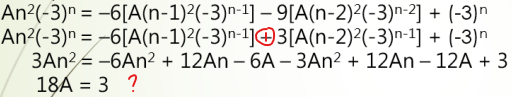The first is a sign error, and the second is unexplainable, as very little should have canceled. But when you fix the sign error, you should get a simple result for A, and the rest of the work should lead to a correct solution.

Here is what his work would have looked like, solving for A:

Putting the trial solution $$p_n=An^2(-3)^n$$ into the recurrence relation $$p_n=-6a_{n-1}-9a_{n-2}+(-3)^n$$ and dividing by $$(-3)^n$$, we get  $$An^2(-3)^n=-6A(n-1)^2(-3)^{n-1}-9A(n-2)^2(-3)^{n-2}+(-3)^n\\ An^2(-3)^n=2A(n^2-2n+1)(-3)^n-A(n^2-4n+4)(-3)^n+(-3)^n\\ An^2=2A(n^2-2n+1)-A(n^2-4n+4)+1\\ An^2=2An^2-4An+2A-An^2+4An-4A+1\\ An^2=An^2-2A+1$$ Setting coefficients of like terms equal, $$A=A\\ 0=-2A+1$$ so that $$A=\frac{1}{2}$$.

If this hadn’t worked, I would have tried a complete polynomial of degree 2, $$a_n=(An^2+Bn+C)(-3)^n$$, as he had initially suggested, in order to provide enough degrees of freedom to solve the resulting equation.

Combining the general and particular solutions, and using B and C rather than his alphas, we have $$a_n=(B+Cn)(-3)^n+\frac{1}{2}n^2(-3)^n$$ Setting $$a_0=2$$ and $$a_1=3$$, we get the system of equations $$(B+C\cdot0)(-3)^0+\frac{1}{2}0^2(-3)^0=2\\ (B+C\cdot1)(-3)^1+\frac{1}{2}1^2(-3)^1=3$$ which reduces to $$B=2\\ -3(B+C)+\frac{-3}{2}=3$$ so that $$B=2$$ and $$C=-\frac{7}{2}$$. The solution, therefore, is $$a_n=(2-\frac{7}{2}n+\frac{1}{2}n^2)*(-3)^n$$

Here is my check: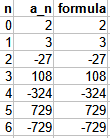## 3: First order again

While we were discussing that second problem, he had also introduced a third, with a different kind of non-homogeneous part:

Find the explicit formula for an = an−1 + n2n + 1 for n ≥ 1 with initial conditions a0 = 1.

What is the particular solution?

I try (An + B)2n + Cn + D, but it seems not to work.

Here he is correctly using the complete polynomial $$An+B$$ rather than just $$An$$. I confirmed his plan:

As for an = an−1+n2n + 1, the particular solution does have the form you suggest, (An + B)2n + Cn + D (though the constant term D will not be determined until later), and the same general method you used before should work. If you show your work again, I can look for an error. (Don’t forget to check your own work for the kinds of errors we’ve already found!)

Now Aaron showed work:

Find the explicit formula for an = an−1 + n2n + 1 for n ≥ 1 with initial conditions a0 = 1.

Characteristic equation is r − 1 = 0

Characteristic root is r = 1

Let an = gn + pn where gn = α(1)n = α and pn= (An+ B)2n + Cn + D

Subs. pn= (An + B)2n + Cn + D into the recurrence relation,

(An+ B)2n + Cn + D = (A(n-1)+ B)2n-1 + C(n-1) + D + n2n + 1

2(An+ B)2n-1 + Cn + D = (An – A + B)2n-1+ 2n2n-1 + Cn – C + D + 1

2A = A+ 2n, 2B = –A + B, C = 0, D = D +1

A = 2n, B = –2n, D = 0

Hence, the particular solution pn = (2n2 – 2n)2n

an = α + (2n2 – 2n)2n

a0 = α + (2(0)2 – 2(0))20

1 = α

So, the explicit formula is an = 1+ (2n2 – 2n)2n.

Your A and B can’t depend on n; they must be constants. So as soon as you got A = 2n, you should have stopped and looked for an error.

I don’t see how you made this step:

2(An + B)2n-1 + Cn + D = (An – A + B)2n-1+ 2n2n-1 + Cn – C + D + 1

2A = A + 2n, 2B = –A + B, C = 0, D = D +1

I would write more steps, like this:

2(An + B)2n-1 + Cn + D = (An – A + B)2n-1+ 2n2n-1 + Cn – C + D + 1

2(An + B)2n-1 = (An – A + B)2n-1+ 2n2n-1 – C + 1

His work was actually quite close, apart from getting the n where it shouldn’t be:

I suspect you may not have been taught this quite clearly enough: You are looking for coefficients that make an equation an identity, not just solving for expressions in terms of n. This is much like what we do in partial fractions, for example.

Now you can expand the entire equation like this, and treat the powers of 2 as if they were another variable:

2(An + B)2n-1 + Cn + D = (An – A + B)2n-1+ 2n2n-1 + Cn – C + D + 1

2An2n-1 + 2B2n-1 + Cn + D = An2n-1 – A2n-1 + B2n-1+ 2n2n-1 + Cn – C + D + 1

so that equating like coefficients leads to this system of equations:

2A = A + 2 → A = 2

2B = -A + B → B = -A = -2

C = C

D = -C + D + 1 → C = 1

D is left undetermined for now.

This is acceptable, because the general solution is itself a constant, D, which will be dealt with in the final work. We could have just omitted D in the particular solution.

Let’s finish it:

Our combined solution is $$a_n=(An+B)2^n+Cn+D=(2n-2)2^n+n+D$$. Setting $$n=0$$, we get $$(2\cdot0-2)2^0+0+D=1\\D-2=1\\ D=3$$ so the final formula is $$a_n=(2n-2)2^n+n+3$$. Here is the table I made to check this: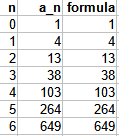## 4: Third order with a twist

Now he sent two versions of a fourth problem. Here is the first, with a correct solution:

Find the explicit formula for an+3 7an+2 + 16an+1 12an = n4n with initial conditions a0 = 2, a1 = 0 and a2 = 5.

Characteristic equation is r3 − 7r2 + 16r – 12 = 0

(r – 2)(r – 2)(r – 3) = 0

Characteristic roots are r = 2 (repeated root) and 3

Let an = gn + pn where gn = (α1 + α2n)(2)n + α3(3)n and pn= (An + B)(4)n.

Subs. pn= An + B into the recurrence relation,

(A(n+3) + B)(4)n+3= 7[(A(n+2) + B)(4)n+2] −16[(A(n+1) + B)(4)n+1] +12[(An + B)(4)n]+ n(4)n

64(An + 3A + B)(4)n= 16(7An +14A + 7B)(4)n− 64(An + A + B)(4)n+(12An +12B)(4)n + n(4)n

64A = 112A − 64A + 12A + 1, 192A + 64B = 224A + 112B − 64A − 64B + 12B

A =1/4, B = −2

Hence, the particular solution pn = (¼ n – 2)(4)n

an = (α1 + α2n)(2)n + α3(3)n +(1/4 n – 2)(4)n

a0 = (α1 + α2(0))(2)0+ α3(3)0+(1/4(0)- 2)(4)0, –2= α1 + α3− 2— (1)

a1 = (α1 + α2(1))(2)1+ α3(3)1+(1/4(1)- 2)(4)1, 0= 2α1 + 2α2 + 3α3 − 7— (2)

a2= (α1 + α2(2))(2)2+ α3(3)2+(1/4(2)- 2)(4)2, 5 = 4α1 + 8α2 + 9α3 − 24 — (3)

α1 = −1, α2 = 3 and α3= 1

So, the explicit formula is an = (3n − 1)(2)n + (3)n +(1/4 n −2)(4)n.

He included a successful check; here is mine: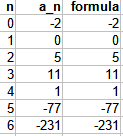He gave a second problem; it appears the same apart from the subscripts, which this time go from $$n$$ to $$n-3$$ rather than from $$n+3$$ to $$n$$:

Is this the same question as above?

Find the explicit formula for an 7an-1 + 16an-2 12an-3 = n4n with initial conditions a0 = 2, a1 = 0 and a2 = 5.

Characteristic equation is r3 − 7r2 + 16r – 12 = 0

(r – 2)(r – 2)(r – 3) = 0

Characteristic roots are r = 2 (repeated root) and 3

Let an = gn + pn where gn = (α1 + α2n)(2)n + α3(3)n and pn= (An + B)(4)n .

Subs. pn= An + B into the recurrence relation,

(An + B)(4)n= 7[(A(n-1) + B)(4)n-1 ] −16[(A(n-2) + B)(4)n-2 ] +12[(A(n-3) + B)(4)n-3]+ n(4)n

64(An + B)(4)n= 112(An – A + B)(4)n− 64(An – 2A + B)(4)n+12(An – 3A +B)(4)n + 64n(4)n

Comparing the coefficient of n, Comparing the constant,

64A = 112A − 64A + 12A + 64, 64B = −112A + 112B + 128A− 64B − 36A + 12B

A =16 B = −80

Hence, the particular solution pn = (16n – 80)(4)n

an = (α1 + α2n)(2)n + α3(3)n +(16n – 80)(4)n

a0 = (α1 + α2(0))(2)0+ α3(3)0+(16(0) – 80)(4)0, –2= α1 + α3− 80— (1)

a1 = (α1 + α2(1))(2)1+ α3(3)1+(16(1) – 80)(4)1, 0= 2α1 + 2α2 + 3α3 − 256— (2)

a2= (α1 + α2(2))(2)2+ α3(3)2+(16(2) – 80)(4)2, 5 = 4α1 + 8α2 + 9α3 − 768 — (3)

α1 = 17, α2 = 39/2 and α3= 61

So, the explicit formula is an = (17+39/2 n)(2)n + 61(3)n +(16n – 80)(4)n.

Why the answers are different? Why I cannot check for it?

Your solution to the first problem here, an = (3n − 1)(2)n + (3)n +(1/4 n −2)(4)n, looks correct when I check it in a spreadsheet.

Here is my check: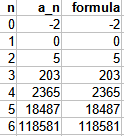The second problem, an−7an-1+16an-2−12an-3 = n4n, is different from the first, because if you replace n+3 in the first problem with n, the RHS becomes (n-3)4n-3.

I get the same result as you for the second problem, and it passes my check.

So the first problem is really equivalent to $$a_n−7a_{n-1}+16a_{n-2}−12a_{n-3} = (n-3)4^{n-3}$$ which is not this second problem.

### 3 thoughts on “Non-homogeneous Recurrence Relations”

1.In solving linear nonhomogeneous recurrence relation given in an + 9an-1 + 20an-2 + F(n) if F(n) = (n^2-n+1)5^n how we can do?

1.Hi, Lami.

What you wrote is not an equation; perhaps you meant a_n + 9a_{n-1} + 20a_{n-2} = (n^2-n+1)5^n.

Since this is a new question, rather than a comment, you should submit it as a question through our Ask a Question link. Please make sure you copy the problem completely and correctly; an image will be ideal. Also, we’ll want to see whatever work you have done, so we can see what help you need.

What troubles me (and perhaps you) is that 5 is not a root of the characteristic equation, though (if I corrected it properly) -5 is. I don’t want to work on it without being sure of the problem.

This site uses Akismet to reduce spam. Learn how your comment data is processed.